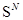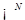## 2010

1. M. J. Kitchen, D. M. Paganin, K. Uesugi, B. J. Allison, R. A. Lewis, S. B. Hooper and K.M. Pavlov. X-ray phase, absorption and scatter retrieval using two or more phase contrast images, Optics Express 18 (2010) 19994-20012.
2. D. Cao, S. Peng and S. Yan, Multiplicity of solutions for the plasma problem in two dimensions. Adv. Math. 225 (2010) 2741-2785.
3. F. Cirstea and Y. Du, Isolated singularities for weighted quasilinear elliptic equations, J. Functional Anal., 259 (2010) 174-202.
4. Y. Du and Z. Lin, Spreading-vanishing dichotomy in the diffusive logistic model with a free boundary, SIAM J. Math. Anal., 42 (2010) 377-405.
5. Y. Du and Hiroshi Matano, Convergence and sharp thresholds for propagation in nonlinear diffusion problems, J. European Math. Soc., 12 (2010) 279-312.
6. J. Wei and S. Yan, On a stronger Lazer-McKenna conjecture for Ambrosetti-Prodi type problems, Ann. Sc. Norm. Super. Pisa Cl. Sci. 9 (2010) 423-457.
7. C.-S. Lin and S. Yan, Bubbling solutions for relativistic abelian Chern-Simons model on a torus, Comm. Math. Phys., 297 (2010) 733-758.
8. D. Cao and S. Yan, Infinitely many solutions for an elliptic problem involving critical Sobolev growth and Hardy potential, Calc. Var. Partial Differential Equations, 38 (2010) 471-501.
9. L. Wang, J. Wei and S. Yan, A Neumann problem with critical exponent in nonconvex domains and Lin-Ni's conjecture, Trans. Amer. Math. Soc., 362 (2010), 4581-4615.
10. J. Wei and S. Yan, Infinitely many solutions for the prescribed scalar curvature problem on, J. Funct. Anal, 258 (2010) 3048-3081.
11. J. Wei and S. Yan, Infinitely many positive solutions for the nonlinear Schrödinger equations in, Calc. Var. Partial Differential Equations 37 (2010) 423-439.
12. J. Schmalz, G. Schmalz, T. Gureyev, and K. Pavlov, On the derivation of the Greens function for the Helmholtz equation using generalized functions, Am. J. Phys., 78 (2010) 181-186.
13. B. Bleile, Poincare duality pairs of dimension three, Forum Math., 22 (2010) 277-301.
14. C. Albert, B. Bleile and J. Frohlich, Batalin-Vilkovisky integrals in finite dimensions, J. Math. Phys., 51 (2010) 015213.